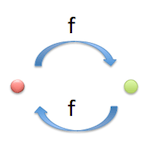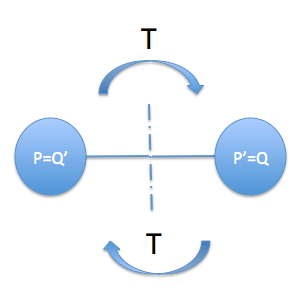# What is an involution in geometry?In geometry, we speak often with terms that, in some cases, they are not sufficiently important in everyday language. This leads to create barriers in the interpretation of some simple concepts.

One of the terms that I have been asked several times in class is the of “Involution”. We define the involution.

?What is an involution?

We will seek a first approximation in the Diccionario de la Lengua Española

“Setback in the progress or evolution of a process” 

Latin origin comes from “Involutio”, “Involutionis”, meaning “wrap”, “Turn one thing into your interior”.

We see that the term indicates a contrary operation that is performed on itself therefore.

What is an involution in mathematics?

If we apply a transformation f an element x of a set X get its transformed x'. This can be expressed as f(x)= x ’.

An involution is a transformation which to apply again the transformation to the previous transformed element gets the starting element

namely:

### f(f(x))= xWhat is an involution in geometry?

In geometry, we work with graphic representations of mathematical elements. We can find for each point, straight or level of their models a mathematical notation that characterizes him, so in principle we should not make any differentiation with regard to the definition given above.

However the elements that we use, even being abstract, they can be manipulated as specific entities (the points of a geometric figure, for example) for what it may be worth talking of “involutionary transformations” to get closer to the concept in graphic operations.

To conclude this short introduction to the concept of involution we may discuss about some examples that clarify us this idea.

A simple involutional transformation is the symmetry, both the axial and power plants. If we transform a point P in P’ using symmetry, It is immediate to see that again applying the transform to P ’, calling him Q, the mutated Q’ matches the original element P.We must not interpret as a reverse transformation involution, Now this last can exist to transform anyone who non-involutive.

For example, There is an inverse function that is different and the same module address but reverse translation in a translation. Translation is not involutive already that if transformed a point P in P’ and we come back to apply the same translation to P’ We don't get the point of initial P.

Some geometric transformations are not involutive and in general may be in any particular case. A turn is not involutional, But if the rotation angle is 180 ° if it will be.

See especially the application of these concepts in projective transformations, studying series and bundles of first and second order in involution.

 Manual of the Vox Spanish language dictionary. © 2007 Editorial Larousse, S.L.